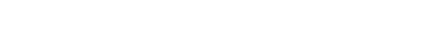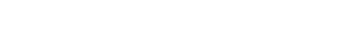## Physics Paper 2 Questions and Answers - Baringo North Joint Evaluation Mock Exams 2022

INSTRUCTIONS TO CANDIDATES

• This paper consists of two sections A and B
• Answer ALL the questions in sections A and B in the spaces provided.
• All workings must be clearly shown. Mathematical tables and silent electronic calculators may be used.
Take h= 6.63 x 10-34 Js

SECTION A: 25 MARKS
Answer all questions in this section

1. Figure 1 shows two mirrors inclined at an angle of 30o to each other. A ray of light is incident on one mirror as shownFig. 1
Sketch the path of the ray to show its reflection on the two mirrors (2mks)
2. Figure 2 below shows two pith balls A and B hanging from nylon threads and brought close to negatively charged rod. Pith ball A is uncharged while B is charged.Fig. 2
It is observed that both pith balls A and B are attracted to the charged rod.
1. State the charge on B (1mk)
2. Explain the reason why pith ball A is attracted (1mk)
3. Show the charge distribution on the pith balls (1mk)
3. Figure 3 shows the path of a ray of light CB passing from glass to air.Fig. 3
Complete the diagram to show the path of the ray of light AB after it emerges from the glass showing the angle calculated. (3mks)
4. Figure 4 shows water waves moving towards barrier.Fig. 4
1. State the property of wave under investigation. (1mk)
2. Show the emergence of the reflected wave after passing the opening (1mk)
3. State why passing of light through narrow opening is a very rare phenomenon (1mk)
5. Figure 5 shows an object in front of a concave mirror and its image.Fig. 5
Locate position of its principal focus and label it as F (2mks)
6. Figure 6 shows a trace obtained from a CRO with the time base switched on.Fig. 6
Draw a circuit diagram that can be used to produce the wave above (2mks)
7. A boy opened up a used-up dry cell and found the following:
1. The zinc casing was ‘eaten away’
2. The cell was watery
Name the cell defect (1 mk)
8. State two uses of microwaves (2mks)
9. Figure 7 shows a wave profile.Fig. 7
Determine the frequency of the wave (3mks)
10. Below is a nuclear reaction.2. Determine the value of X and Y (1mk)
11. Figure 8 shows a bar of soft iron placed near a magnet.Fig. 8
On the same diagram, sketch the magnetic field pattern due to the set up (2mks)

SECTION B. (55 MARKS)
Answer all questions in this section

1.
1. Figure 9 shows an object, a screen and light sources X, Y and Z.Fig. 9
1. Complete the diagram to show the formation of a shadow (2mks)
2. State one property of the object that makes it possible for its shadow to be formed   (1mk)
3. Explain why it is impossible to obtain a sharp edged shadow of the object (1mk)
2.
1. The length of a pinhole camera is 20cm. Determine the height of a sloppy building 300m away from a pinhole camera which forms an image 2.5cm high on the screen of the camera. (3mks)
2. A student decided to use a nail to make the hole of the camera mentioned above. State two possible characteristics of the image formed (2mks)
2. Figure 10 shows an X-ray tube.Fig. 10
1. Explain the use of part labelled B and C (2mks)
2. Explain how the X-rays are produced. (2mks)
3. Why is it necessary to maintain a vacuum inside the tube? (1mk)
3.
1. What is meant by the term electromotive force of a cell? (1mk)
2. Battery of e.m.f. 12 V supplies a current of 10 A flows through it for 5 minutes. Determine the amount of electrical energy produced. (2mks)
3. Figure 11 shows a graph of resistance against reciprocal of current. Use it to answer the questions that follow.Fig. 11
1. Find the internal resistance, r. (2mks)
2. Determine the e.m.f, E of the cell. (2mks)
3. Draw a circuit diagram to show the set-up used to generate the above graph (2mks)
4.
1. Draw a circuit diagram to show how a 12V battery may be used to operate efficiently 3 headlamp bulbs each marked 6V, 24W. (2mks)
2. Calculate the current supplied by the battery in (d) above. (2mks)
3. Calculate the total effective resistance of the bulbs in (i) above. (2mks)
4.
1. State what is meant by the term electromagnetic induction. (1mk)
2. Figure 12 shows a simple electric generatorFig. 12
1. ​Name the parts labelled X, Y and Z. (3mks)
2. Show the direction of induced current along BC (1mk)
3. Sketch on the axes provided, a graph to show how the magnitude of the potential difference across R, changes with the time t for one rotation of the coil when it starts from horizontal position (1mk)4. ​State the effect of using a magnet with curved poles as shown in the diagram (2mks)
3. In a transformer, the ratio of primary turns to the secondary turns is 2:20. A current of 500 mA flows through a 400 ohms resistor in the secondary circuit. Assuming that the transformer is 100% efficient, determine:
1. the secondary voltage (2mks)
2. the primary voltage (2mk)
3. the primary current. (2mks)
5.
1. Figure 13 shows a graph of photoelectric current against frequency. Use it to answer questions that follow.Fig. 13
1. What does the term fo stand for in the graph above? (2mks)
2. Explain what the graph shows in terms of photoelectric emission. (2mks)
2. The work function of a metal is 6.4 x 10-19 J.
1. Explain what is meant by the term work function (1mk)
2. Light with a frequency of 1.2 x 1015 Hz is shone onto the metal surface. Find out whether or not the photons of this light will cause the photoelectric effect to take place. (3mks)
3. The light source is now replaced with a light source which produces light with a frequency of 1.5 x 1015 Hz. The photons from this source contain more energy than is required to release electrons.
1. Determine the extra energy available after the electron has been released. (3mks)
2. What is the extra energy calculated above converted to? (1mk)## MARKING SCHEME

1.2.
1. Positive
2. Positive charges are induce on the surface/negative charges are repelled hence the attraction.
3.3. n =    1
sin C
n =       1
sin 43.6
n = 1.581
1.581 = sin 30
sin r
r = 18.54°4.
1. Diffraction
2.3. Light waves have much shorter wavelength
5.6.7. Local action
8.
• Used for cooking
9. f = 1/T
1
0.16
= 6.25 Hz
10.
2. X= 228    Y= 88
11.12.
1.
1.2. It is opaque/does not allow light to pass through
3. it acts as an extended source
2.
1. hi = V
ho   U
ho = 0.025 x 300
0.2
= 37.5 m
2.
• Blurred
• Brighter
13.
1.
• B: Lead shield: to absorb stray X-rays
• C: step-up transformer- to supply power
2. Filament is heated and then heats the cathode with sufficient energy. Electrons are then emitted from the cathode by process of thermionic emission. The fast moving electrons are accelerated and focused to a target where they are stopped with only about 2 % of the energy converted to X-rays.
3. To ensure that the electrons emitted do not lose energy by colliding with foreign materials e.g. air molecules.
14.
1. the voltage across the terminals of a cell in an open circuit
2. H = 12 x 10 x 5 x60
= 36000 J.
3.
1. internal resistance r = y-intercept
= 0.5 Ω
2. R= E − r
I
3 − 1.5
0.7 − 0.4
= 5 V
3.4.
1.2. I= P
V
= 6V
4A
= 1.5 Ω
RT = R/3
RT = 1.5/3
= 0.5 Ω
15.
1. the production of induced emf which causes induced current to flow by changing magnetic flux linkage in a coil.
2.
1.
• X: Brushes
• Y: Slip rings
• Z: Turns of the coil
2.3.4. it creates concentric loops which concentrate magnetic fields/flux
3.
1.  500 x 400
1000
= 200V
2. 200 x 2
20
= 20 V
3. Ip = 0.5 x 20
2
V= 5 A
16.
1.
1. threshold frequency- the minimum frequency of an electromagnetic radiation required to dislodge an electron from the metal surface.
2. at threshold frequency photoelectric emission takes place. The magnitude of photo current depends on the frequency. As the frequency increases the magnitude of photocurrent increases upto some point.
2.
1. minimum energy require to dislodge an electron from a metal surface.
2. hf = hfo + ½ mvmax2
hf = 6.63 x 10-34 x 1.2 x 1015
= 7.956 x 10-19
hf is greater than the work function hence photoemission occurs.
3.
1. K.E.
(1.5 x 1015 x 6.63 x 10-34) -6.4 x 10-19
3.545 x 10-19 J
2. Maximum kinetic energy.

• ✔ To read offline at any time.
• ✔ To Print at your convenience
• ✔ Share Easily with Friends / Students